# (Solved Homework): Question: In this assignment, you are asked to write the definition for a rectangle class and test it with the test

Question: In this assignment, you are asked to write the definition for a rectangle class and test it with the test program provided. The description of the rectangle class and the driver program are given on the attached file rectangle.cpp. Download the rectangle.cpp and without removing or changing any of the member functions or member variables of this class, complete 10 member functions bodies such that your program produces exact output as provided. You should keep the class definition and the main function in the same source file, and you are not allowed to use global variables. Your program should be well commented and in particular, begin with a series of comments specifying your name and lab number. (You should not change class definition or main function. Just complete the rest of the work.) rectangle.cpp below: #include using namespace std; class rectangle { public: rectangle(int len, int wid); //Parameterized Constructor rectangle(); //Default Constructor; Default lenght is 20 and default width is 10 void setWidth(int newWid); //Mutator function void setLength(int newLen);//Mutator function int getWidth() const;//Accessor function int getLength() const;//Accessor function void printRectangle(); //Print function int getArea();//Find Area; hint: Area is width * length int getPrimeter();//Find Perimeter; hint: Perimeter is (width + length)*2 bool equals(const rectangle& otherRectangle) const; //Comparison function private: int length; //variable to store length int width; //variable to store width }; //Start your class functions codes from here! // Driver program for the rectangle class int main() { rectangle s1; rectangle s2(5, 20); rectangle s3; //Display rectangles cout << “Rectangle s1 “; s1.printRectangle(); cout << “Rectangle s2 “; s2.printRectangle(); cout << “Rectangle s3 “; s3.printRectangle(); // Test Equality if (s1.equals(s2)) cout << “s1 and s2 are equal rectangle!” << endl; else cout << “s1 and s2 are unequal rectangle!” << endl; if (s1.equals(s3)) cout << “s1 and s3 are equal rectangle!” << endl; else cout << “s1 and s3 are unequal rectangle!” << endl; //Display area and perimeter cout << “Area of s1=” << s1.getArea() << endl; cout << “Area of s2=” << s2.getArea() << endl; cout << “Perimeter of s1=” << s1.getPrimeter() << endl; cout << “Perimeter of s2=” << s2.getPrimeter() << endl; return 0; }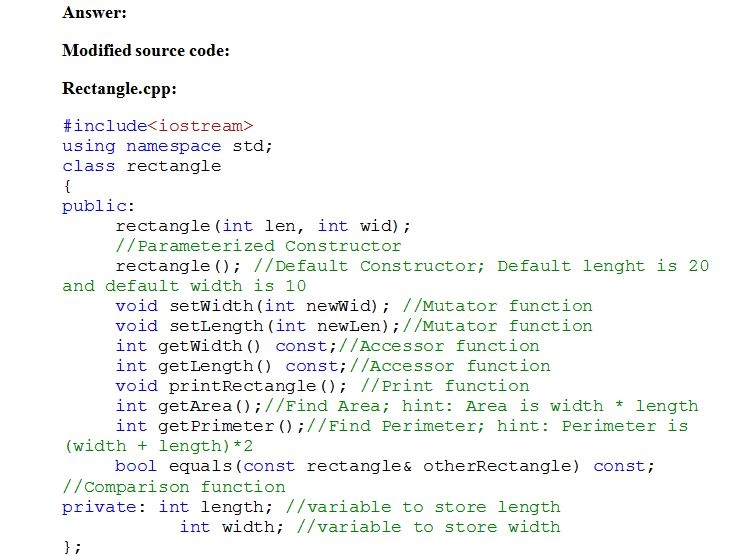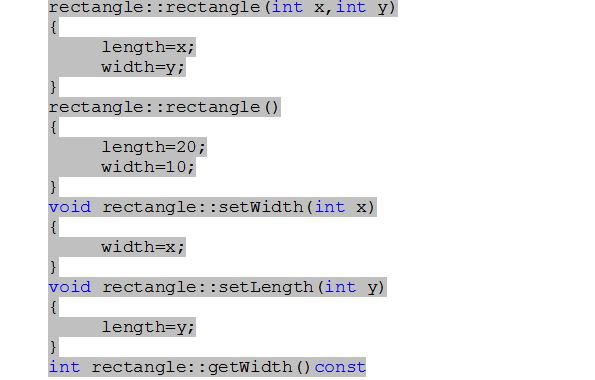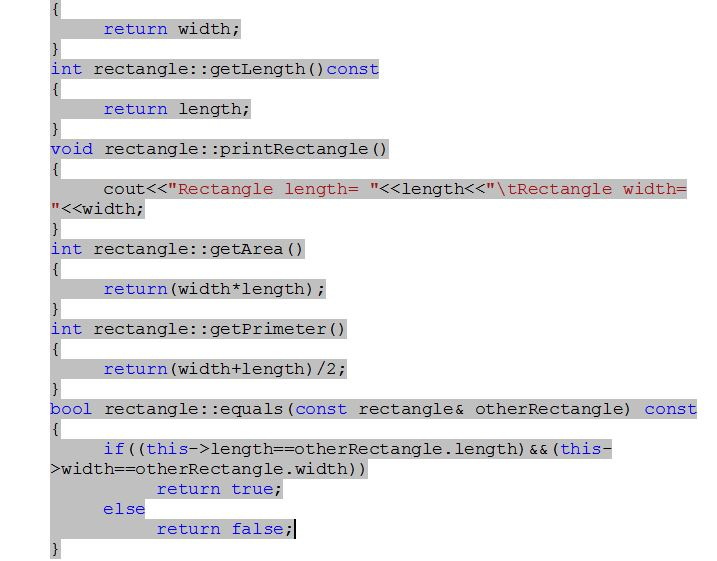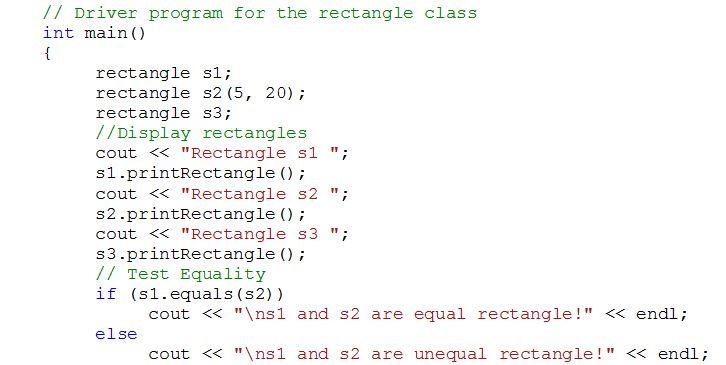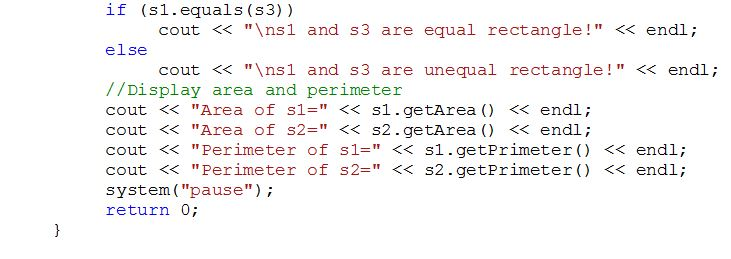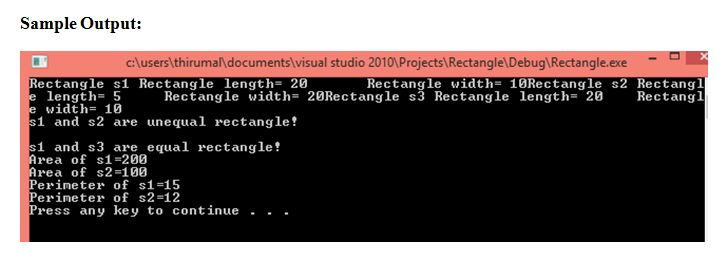Program code:

#include<iostream>
using namespace std;
class rectangle
{
public:
rectangle(int len, int wid);
//Parameterized Constructor
rectangle(); //Default Constructor; Default lenght is 20 and default width is 10
void setWidth(int newWid); //Mutator function
void setLength(int newLen);//Mutator function
int getWidth() const;//Accessor function
int getLength() const;//Accessor function
void printRectangle(); //Print function
int getArea();//Find Area; hint: Area is width * length
int getPrimeter();//Find Perimeter; hint: Perimeter is (width + length)*2
bool equals(const rectangle& otherRectangle) const; //Comparison function
private: int length; //variable to store length
int width; //variable to store width
};
rectangle::rectangle(int x,int y)
{
length=x;
width=y;
}
rectangle::rectangle()
{
length=20;
width=10;
}
void rectangle::setWidth(int x)
{
width=x;
}
void rectangle::setLength(int y)
{
length=y;
}
int rectangle::getWidth()const
{
return width;
}
int rectangle::getLength()const
{
return length;
}
void rectangle::printRectangle()
{
cout<<“Rectangle length= “<<length<<“tRectangle width= “<<width;
}
int rectangle::getArea()
{
return(width*length);
}
int rectangle::getPrimeter()
{
return(width+length)/2;
}
bool rectangle::equals(const rectangle& otherRectangle) const
{
if((this->length==otherRectangle.length)&&(this->width==otherRectangle.width))
return true;
else
return false;
}

// Driver program for the rectangle class
int main()
{
rectangle s1;
rectangle s2(5, 20);
rectangle s3;
//Display rectangles
cout << “Rectangle s1 “;
s1.printRectangle();
cout << “Rectangle s2 “;
s2.printRectangle();
cout << “Rectangle s3 “;
s3.printRectangle();
// Test Equality
if (s1.equals(s2))
cout << “ns1 and s2 are equal rectangle!” << endl;
else
cout << “ns1 and s2 are unequal rectangle!” << endl;
if (s1.equals(s3))
cout << “ns1 and s3 are equal rectangle!” << endl;
else
cout << “ns1 and s3 are unequal rectangle!” << endl;
//Display area and perimeter
cout << “Area of s1=” << s1.getArea() << endl;
cout << “Area of s2=” << s2.getArea() << endl;
cout << “Perimeter of s1=” << s1.getPrimeter() << endl;
cout << “Perimeter of s2=” << s2.getPrimeter() << endl;
system(“pause”);
return 0;
}Order NOW for a 10% Discount
Pages (550 words)
Approximate price: -

Why Us?Top Quality and Well-Researched Papers

All ourbpapers are written from scratch. In fact, Clients who ask for paraphrasing services are highly discouraged. We have writers ready to craft any paper from scratch and deliver quality ahead of time.Our writers keeps you posted on your papers progress - providing you with paper outline/draft. You are also at liberty to communicate directly with your writer.Free Unlimited Revisions

If you think we missed something, send your order for a free revision. You have 10 days to submit the order for review after you have received the final document. You can do this yourself after logging into your personal account or by contacting our support.Prompt Delivery and 100% Money-Back-Guarantee

All papers are always delivered on time, in many cases quite ahead of time. In case we need more time to master your paper, we may contact you regarding the deadline extension. In case you cannot provide us with more time, a 100% refund is guaranteed.Original & Confidential

We use several writing tools checks to ensure that all documents you receive are free from plagiarism. Our editors carefully review all quotations in the text. We also promise maximum confidentiality in all of our services.Our support agents are available 24 hours a day 7 days a week and committed to providing you with the best customer experience. Get in touch whenever you need any assistance.

Try it now!

## Calculate the price of your order

Total price:
\$0.00

How it works?Fill in the order form and provide all details of your assignment.Proceed with the payment

Choose the payment system that suits you most.Our Services

No need to work on your paper at night. Sleep tight, we will cover your back. We offer all kinds of writing services.## Essay Writing Service

No matter what kind of academic paper you need and how urgent you need it, you are welcome to choose your academic level and the type of your paper at an affordable price. We take care of all your paper needs and give a 24/7 customer care support system.An admission essay is an essay or other written statement by a candidate, often a potential student enrolling in a college, university, or graduate school. You can be rest assurred that through our service we will write the best admission essay for you.Editing Support

Our academic writers and editors make the necessary changes to your paper so that it is polished. We also format your document by correctly quoting the sources and creating reference lists in the formats APA, Harvard, MLA, Chicago / Turabian.Revision Support

If you think your paper could be improved, you can request a review. In this case, your paper will be checked by the writer or assigned to an editor. You can use this option as many times as you see fit. This is free because we want you to be completely satisfied with the service offered.